Next: 17.2 Basic Formulas Up: 17. Antidifferentiation Techniques Previous: 17. Antidifferentiation Techniques   Index

# 17.1 The Antidifferentiation Problem

17.1   Definition (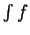or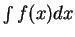.) I am going to use the notationorto denote some arbitrary antiderivative for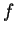on an interval that often will not be specified. This is the same notation that I used previously to denote an indefinite integral for. Although the fundamental theorem of the calculus shows that for nice functions the concepts of antiderivative'' and indefinite integral'' are essentially the same, for arbitrary functions the two concepts do not coincide. For example, let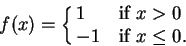Thenhas an indefinite integral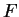where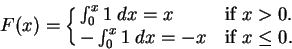Thus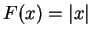. Thenis not an antiderivative for, since we know thatis not differentiable at 0.

I will always try to make it clear whetherrepresents an antiderivative or an indefinite integral in cases where it makes a difference.

The problem of calculating derivatives is straightforward. By using known formulas and rules, you can easily find the derivative of almost any function you can write down. The problem of calculating antiderivatives is much more complicated. In fact, none of the five functions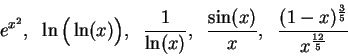(17.2)

have antiderivatives that can be expressed in terms of functions we have studied. (To find a proof of this assertion, see [40, page 37 ff] and .) The first two functions in this list are compositions of functions that have simple antiderivatives, the third function is the reciprocal of a function with a simple antiderivative, and each of the last two functions is a product of two functions with simple antiderivatives. (An antiderivative for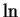will be calculated in (17.25).) It follows that there is no chain rule or reciprocal rule or product rule for calculating antiderivatives. We will see, however, that the chain rule and the product rule for differentiation do give rise to antidifferentiation formulas.

The five functions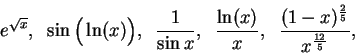(17.3)

which look somewhat similar to the functions in (17.2), turn out to have simple antiderivatives, as you will see in (17.42c), (17.22), (17.7), (17.31f), and (17.41). It is often not easy to tell the difference between a function that has a simple antiderivative and a function that does not.

Many simple functions that arise in applied problems do not have simple antiderivatives. The exercises in this chapter have been carefully designed to be non-typical functions whose antiderivatives can be found.

The Maple instructions for finding antiderivatives and integrals are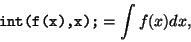and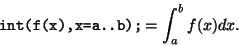I gave the five functions in (17.2) to Maple to antidifferentiate.

The results were:

> int( exp(x^2),x);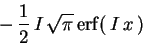> int( ln(ln(x)),x);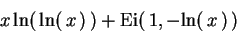> int(1/ln(x),x);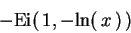> int(sin(x)/x,x);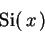> int( ((1-x)^(3/5))/ (x^(12/5)),x);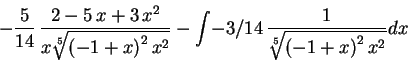In the first four cases, an answer has been given involving names of functions we have not seen before, (and which we will not see again in this course). The definitions of these functions are: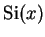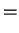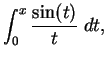(17.4)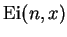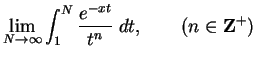(17.5)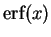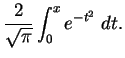(17.6)

In equation (17.4), we assume that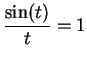when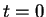. The function Si is called the sine integral. In equation (17.5), Ei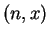makes sense only when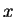is positive. The definition of Eifor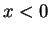involves ideas we have not discussed. The function Ei is called the exponential integral.

The function erf is called the error function. The answer given by Maple for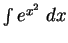involves the symbol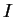. This is Maple's notation for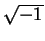. The definition of erf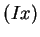makes no sense in terms of concepts we have studied. However you can use Maple to calculate integrals even if you do not know what the symbols mean. The following instructions find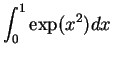:

> int( exp(x^2),x= 0..1);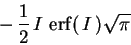> evalf(%);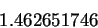Next: 17.2 Basic Formulas Up: 17. Antidifferentiation Techniques Previous: 17. Antidifferentiation Techniques   Index
Ray Mayer 2007-09-07